# 2. Let the components of a stress matrix at a point P in a body be...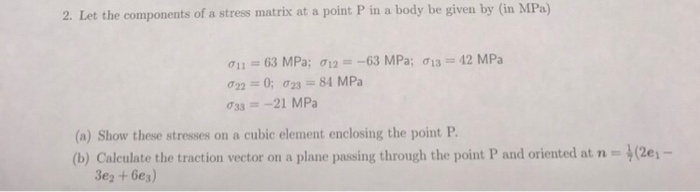2. Let the components of a stress matrix at a point P in a body be given by (in MPa) σ11 = 63 MPa; σ12 =-63 MPa; 013 = 42 MPa ơ33-:-21 MPa (a) Show these stresses on a cubic element enclosing the point P (b) Calculate the traction vector on a plane passing through the point P and oriented at n- 2e1 3e2 +6e3)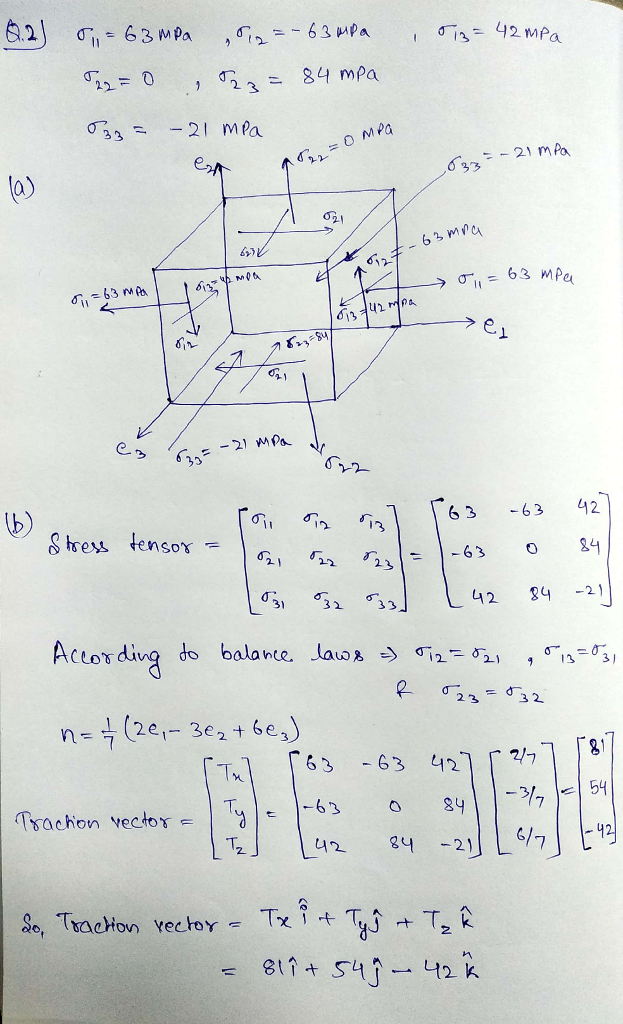#### Earn Coin

Coins can be redeemed for fabulous gifts.

Similar Homework Help Questions
• ### The state of stress at a point on a body is given by the following stress...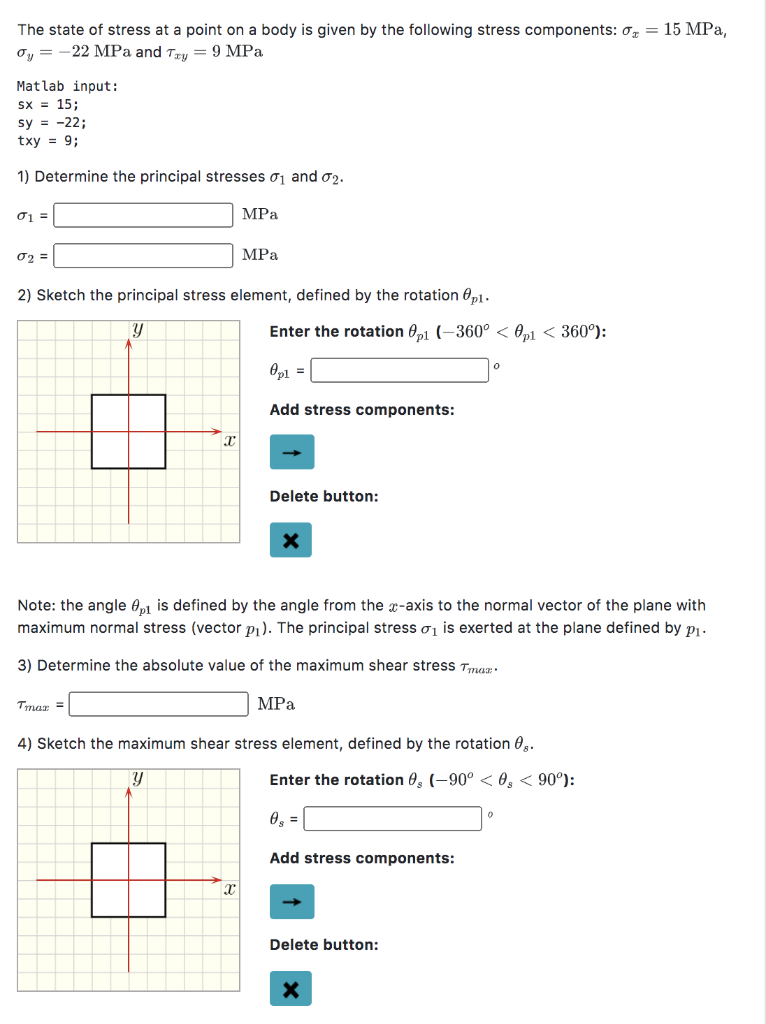The state of stress at a point on a body is given by the following stress components: 0 = 15 MPa, Oy = -22 MPa and Try = 9 MPa Matlab input: sx = 15; sy = -22; txy = 9; 1) Determine the principal stresses 01 and 02. 1 = MPa 02= MPa 2) Sketch the principal stress element, defined by the rotation @pl. y Enter the rotation @pi (-360º < Opl < 360°): Opl = Add stress components:...

• ### Question 5 (1 mark) Attempt 1 In a solid body, the six components of the stress at a point P, are given by: σ,-90 M...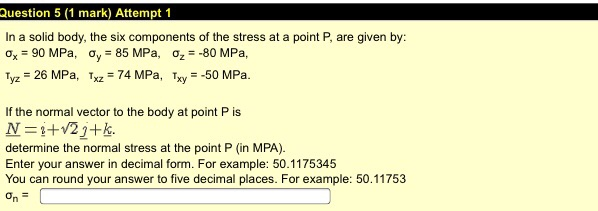Question 5 (1 mark) Attempt 1 In a solid body, the six components of the stress at a point P, are given by: σ,-90 MPa, ơy-85 MPa, σ,--80 MPa. Tyz 26 MPa, Txz74 MPa, Txy50 MPa If the normal vector to the body at point P is determine the normal stress at the point P (in MPA) Enter your answer in decimal form. For example: 50.1175345 You can round your answer to five decimal places. For example: 50.11753 Question 5...

• ### The state of plane stress at a point on a body is represented by the element...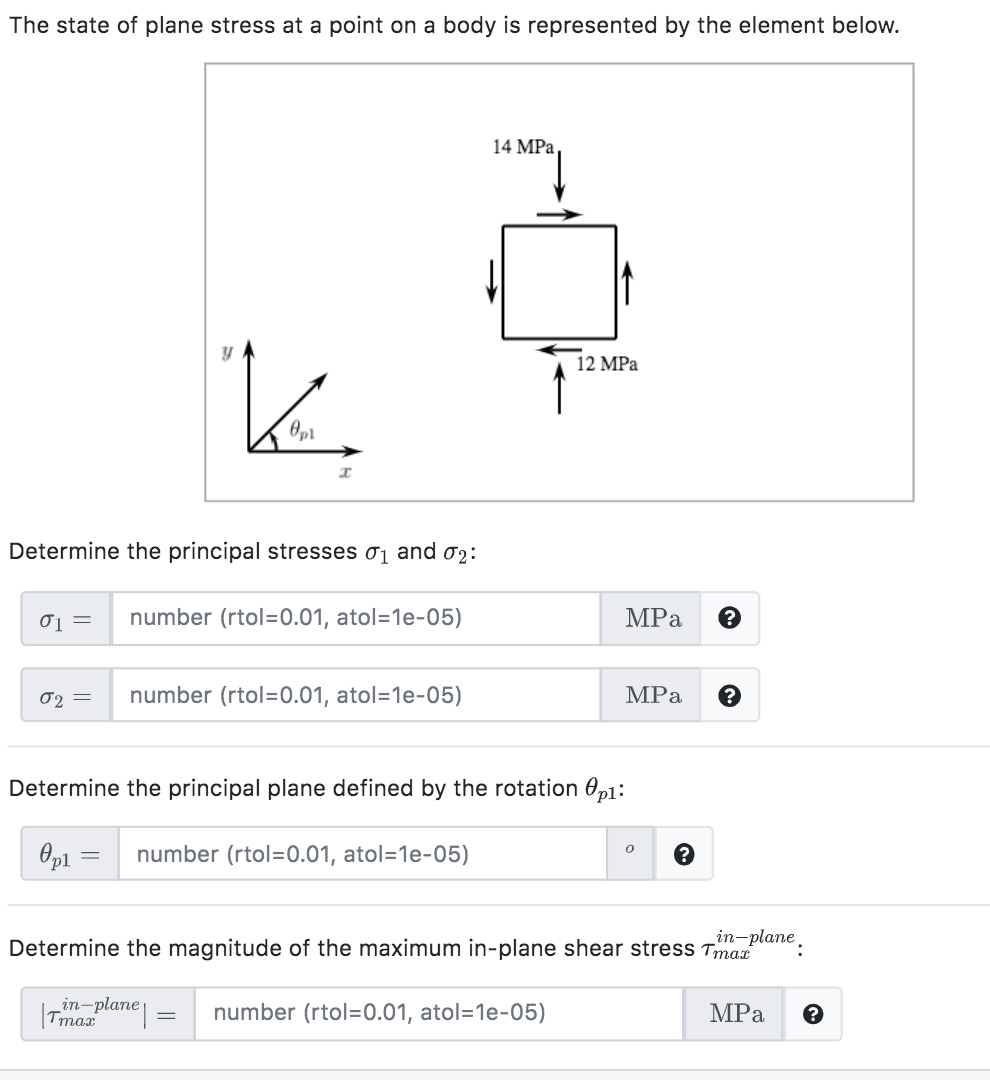The state of plane stress at a point on a body is represented by the element below. 14 MPa y 12 MPa K. Determine the principal stresses 01 and 02: 01 = number (rtol=0.01, atol=1e-05) MPa 02 = number (rtol=0.01, atol=1e-05) MPa ? Determine the principal plane defined by the rotation Opi: 0 number (rtol=0.01, atol=1e-05) Opl in-plane Determine the magnitude of the maximum in-plane shear stress Tmac Terima plane number (rtol=0.01, atol=1e-05) MPa

• ### The state of plane stress at a point on a body is represented by the element...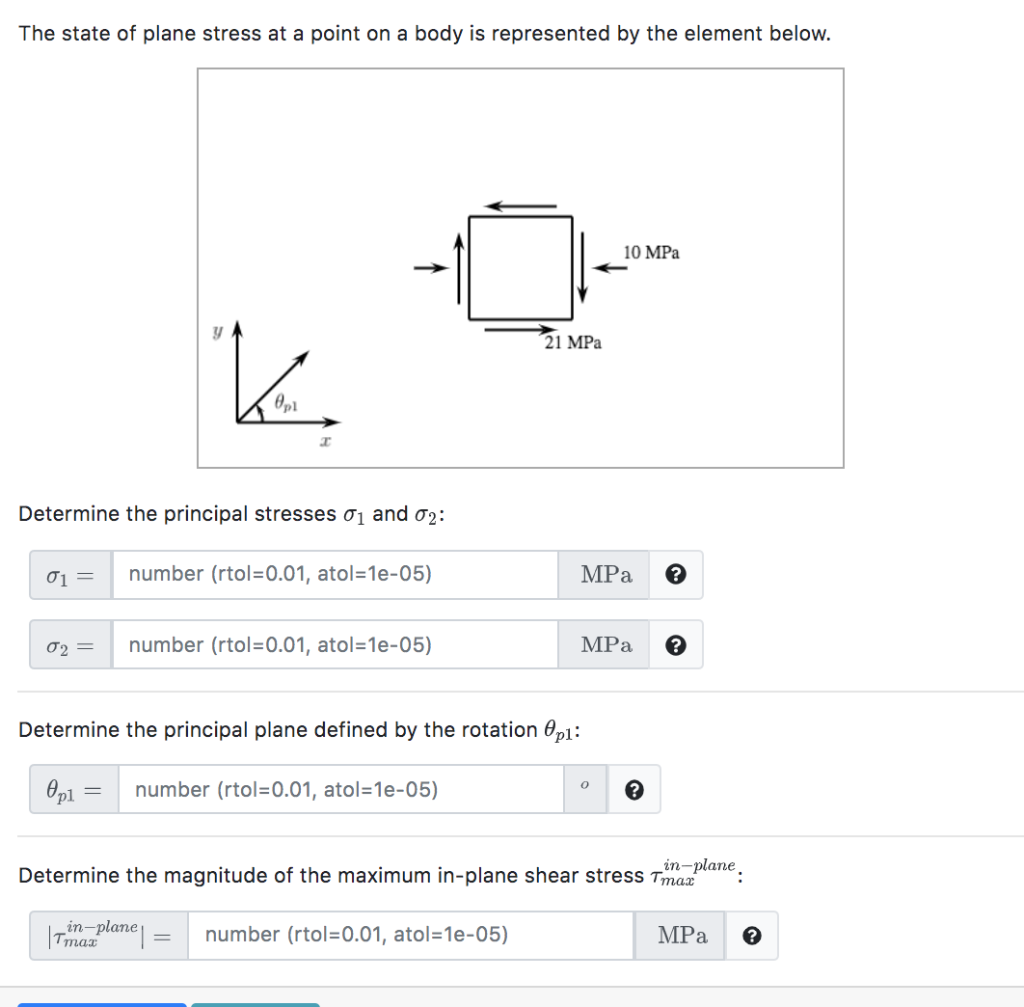The state of plane stress at a point on a body is represented by the element below. 10 MPa → 21 MPa K өр Determine the principal stresses 01 and 02: 01 = number (rtol=0.01, atol=1e-05) MPa 02 = number (rtol=0.01, atol=1e-05) MPa Determine the principal plane defined by the rotation @p1: o Opl = number (rtol=0.01, atol=1e-05) in-plane Determine the magnitude of the maximum in-plane shear stress Tmaz Irina plane number (rtol=0.01, atol=1e-05) MPa

• ### Problem 6 (15 points) The state of plane stress at a point is shown on the...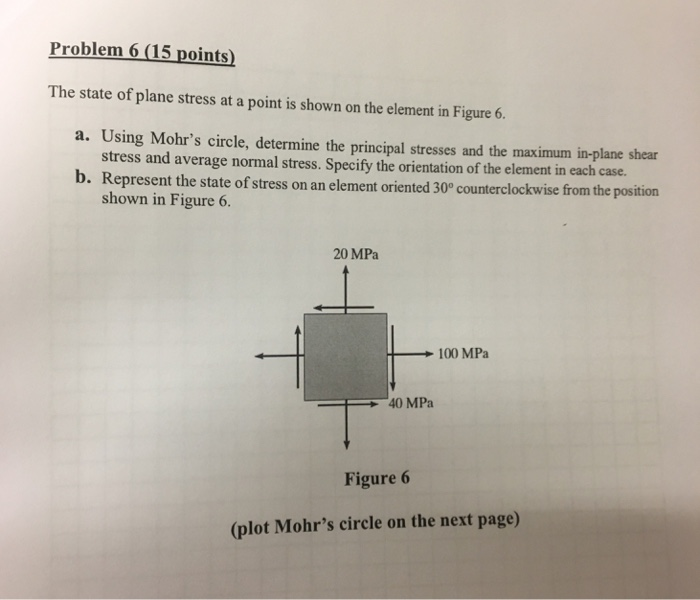Problem 6 (15 points) The state of plane stress at a point is shown on the element in Figure 6. a. Using Mohr's circle, determine the principal stresses and the maximum in-plane shear stress and average normal stress. Specify the orientation of the element in each case. b. Represent the state of stress on an element oriented 30° counterclockwise from the position shown in Figure 6. 20 MPa 100 MPa 40 MPa Figure 6 (plot Mohr's circle on the next...

• ### The state of plane stress at a point on a body can be depicted by the...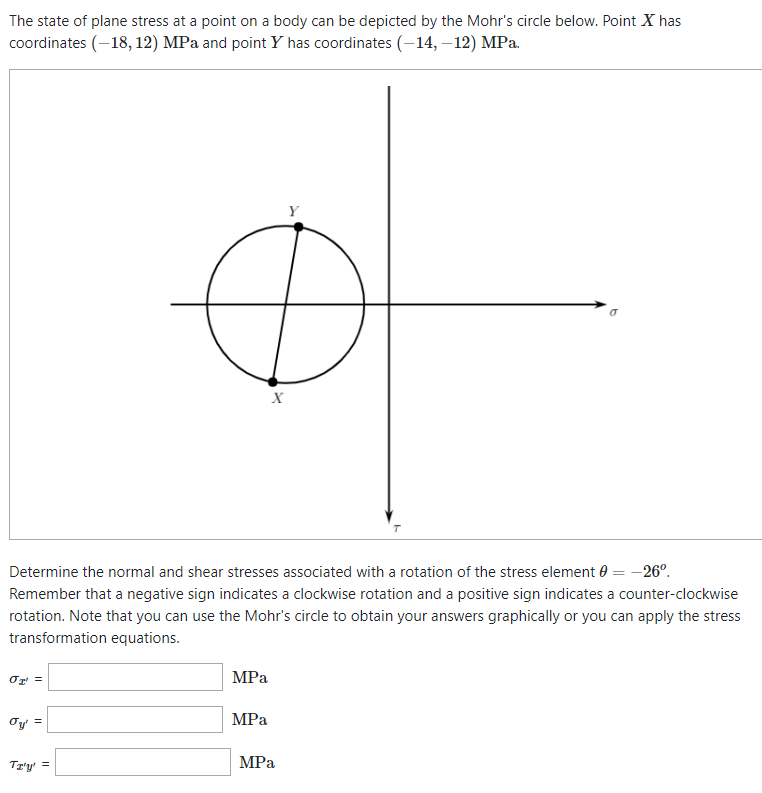The state of plane stress at a point on a body can be depicted by the Mohr's circle below. Point X has coordinates(-18, 12) MPa and point Y has coordinates (-14,-12) MPa Determine the normal and shear stresses associated with a rotation of the stress element θ--26 Remember that a negative sign indicates a clockwise rotation and a positive sign indicates a counter-clockwise rotation. Note that you can use the Mohr's circle to obtain your answers graphically or you can...

• ### The state of plane stress at a point under the surface of the ANKA airplane wing...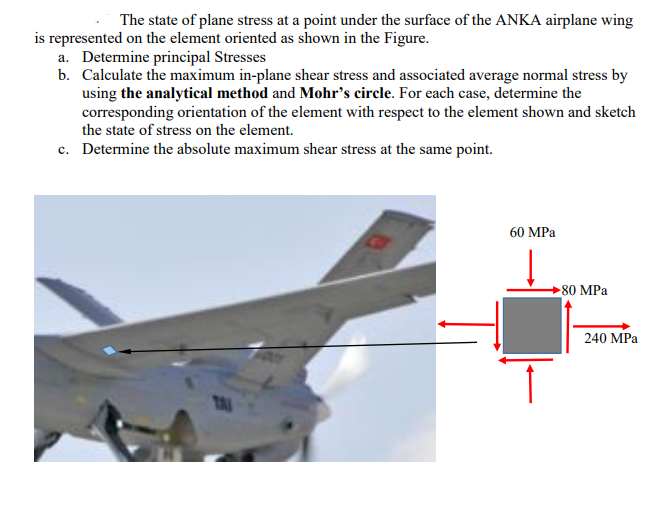The state of plane stress at a point under the surface of the ANKA airplane wing is represented on the element oriented as shown in the Figure. Deternine principal Stresses Calculate the maximum in-plane shear stress and associated average normal stress by using the analytical method and Mohr's circle. For each case, determine the corresponding orientation of the element with respect to the element shown and sketch the state of stress on the element. Determine the absolute maximum shear stress...

• ### The state of stress at a point is shown on the element. Determine (a) the stress components acting on the inclined plan...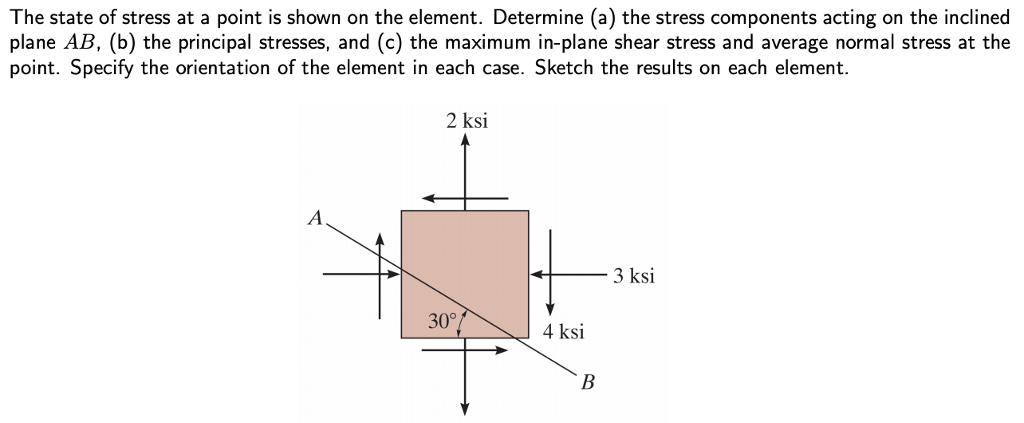The state of stress at a point is shown on the element. Determine (a) the stress components acting on the inclined plane AB, (b) the principal stresses, and (c) the maximum in-plane shear stress and average normal stress at the point. Specify the orientation of the element in each case. Sketch the results on each element. 2 ksi 3 ksi 30° 4 ksi

• ### The state of stress at a point is shown on the element. Determine (a) the stress components acting on the inclined plan...The state of stress at a point is shown on the element. Determine (a) the stress components acting on the inclined plane AB, (b) the principal stresses, and (c) the maximum in-plane shear stress and average normal stress at the point. Specify the orientation of the element in each case. Sketch the results on each element. 2 ksi 3 ksi 30° 4 ksi

• ### your Consider the element in plane stress as shown below. () (2 points) Draw corresponding Mohr's...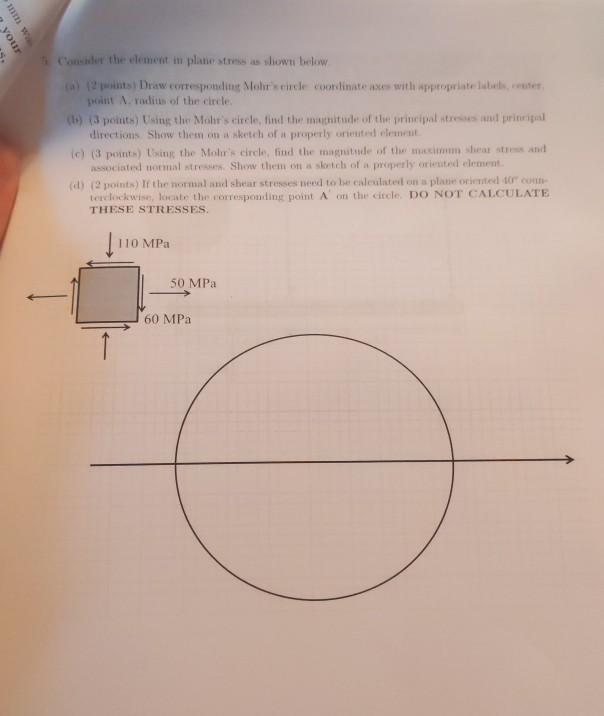your Consider the element in plane stress as shown below. () (2 points) Draw corresponding Mohr's circle coordinate axes with appropriate labels, center point A. radius of the circle (1) (3 points) Using the Mohir's circle, find the magnitude of the principal stresses and principal directions. Show them on a sketch of a properly oriented clement (c) (3 points) Using the Mohr's circle, find the magnitude of the maximun shear stress and associated normal stresses Show them on a sketch...